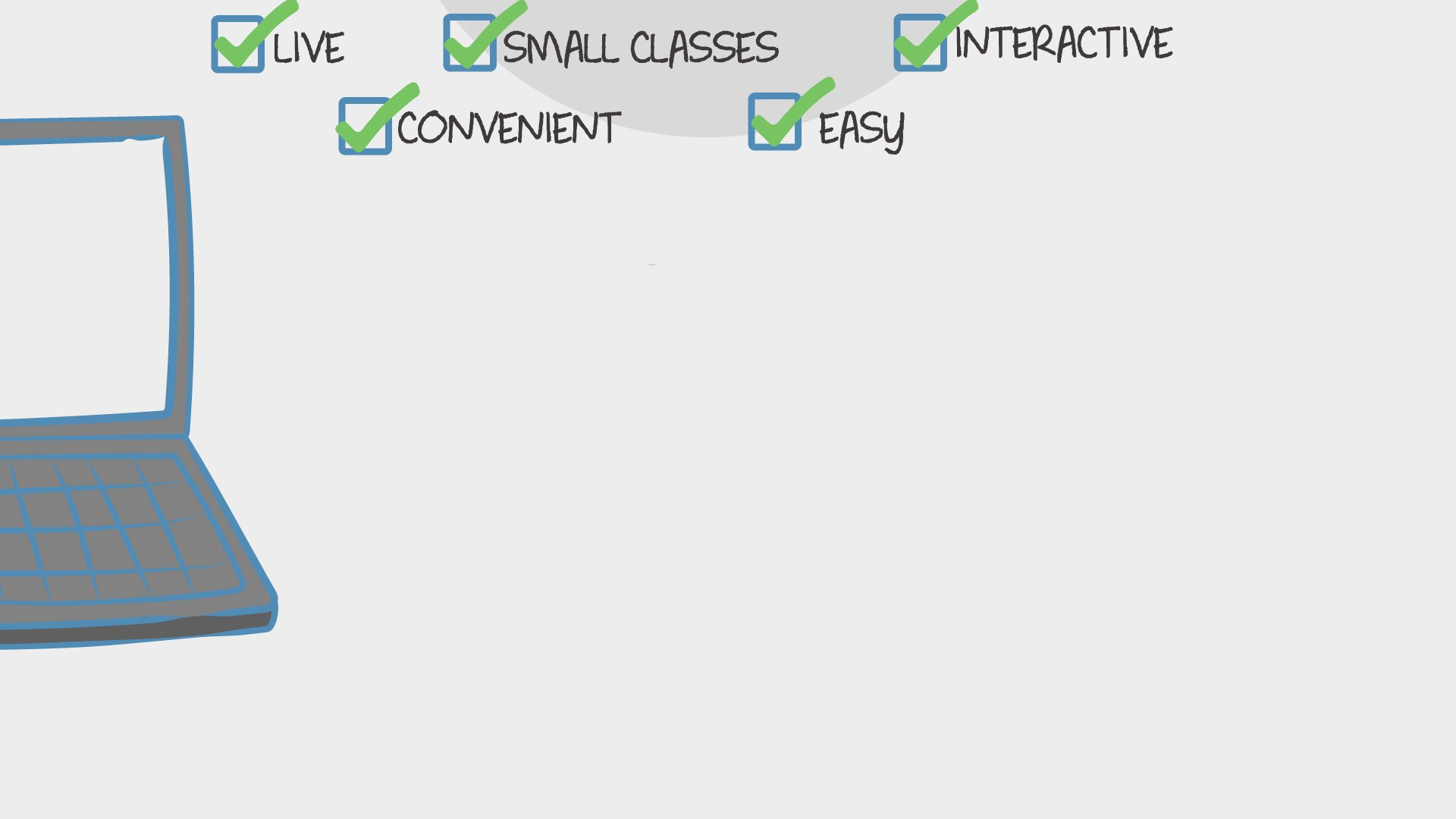Math

## 1st Grade Math (Weeks 17-24)

In this 8 week class we will learn 1st grade, 3rd quarter math. I will be using common core math.
520 total reviews for this teacher
2 reviews for this class
Completed by 11 learners
There are no upcoming classes.per class

over 8 weeks

year olds

#### 3-7

learners per class
per learner - per class

### How does a “Multi-Day” course work?

Meets multiple times at scheduled times
Live video chats, recorded and monitored for safety and quality
Discussions via classroom forum and private messages with the teacher
Great for engaging projects and interacting with diverse classmates from other states and countries### How Outschool Works### There are no open spots for this class.

You can request another time or scroll down to find more classes like this.

## Description

#### Class Experience

```Represent and solve problems involving addition and subtraction.
1.OA.1 Use addition and subtraction within 20 to solve word problems involving situations of adding
to, taking from, putting together, taking apart, and comparing, with unknowns in all positions,
e.g., by using objects, drawings, and equations with a symbol for the unknown number to
represent the problem.
1.OA.2 Solve word problems that call for addition of three whole numbers whose sum is less than or
equal to 20, e.g., by using objects, drawings, and equations with a symbol for the unknown
number to represent the problem.
Understand and apply properties of operations and the relationship between addition and
subtraction.
1.OA.3 Apply properties of operations as strategies to add and subtract. (Students need not use
formal terms for these properties.) Examples: If 8 + 3 = 11 is known, then 3 + 8 = 11 is also
known. (Commutative property of addition.) To add 2 + 6 + 4, the second two numbers can be
added to make a ten, so 2 + 6 + 4 = 2 + 10 = 12. (Associative property of addition.)
1.OA.4 Understand subtraction as an unknown-addend problem. For example, subtract 10 – 8 by
finding the number that makes 10 when added to 8.
1.OA.6 Add and subtract within 20, demonstrating fluency for addition and subtraction within 10.
Use mental strategies such as counting on; making ten (e.g., 8 + 6 = 8 + 2 + 4 = 10 + 4 = 14);
decomposing a number leading to a ten (e.g., 13 – 4 = 13 – 3 – 1 = 10 – 1 = 9); using the
relationship between addition and subtraction (e.g., knowing that 8 + 4 = 12, one knows 12 –
8 = 4); and creating equivalent but easier or known sums (e.g., adding 6 + 7 by creating the
known equivalent 6 + 6 + 1 = 12 + 1 = 13).
Understand place value.4
1.NBT.2 Understand that the two digits of a two-digit number represent amounts of tens and ones.
Understand the following as special cases:
a. 10 can be thought of as a bundle of ten ones—called a “ten.”
b. The numbers from 11 to 19 ```
`Students will need a paper and pencil for each class. Once we start module 3 the students will need a ruler. `
`Learners will not need to use any apps or websites beyond the standard Outschool tools.`
`I will assess the students by observation. I will also provide formal assessments to the parents that they can use if they would like. We will also do mid-assessments and assessments at the end of a module. `
`1 hour 15 minutes per week in class, and maybe some time outside of class.`
`If the students are able to move faster we will move faster through the content. If we need to slow down a bit we will do that also. We will move at the pace of the students in the class. `

## Teacher

`Hi, my name is Madison. I have a Bachelor's of Science Degree in Elementary Education with an emphasis in Social Science and Math. I have a 5 year old son and a two year old daughter. We love to play outside and create art with the things we find... `